Students appreciate clear and concise ICSE S Chand Maths Class 9 Solutions Chapter 18 Surface Area and Volume of 3D Solids Ex 18(A) that guide them through exercises.

## S Chand Class 9 ICSE Maths Solutions Chapter 18 Surface Area and Volume of 3D Solids Ex 18(A)

Question 1.
Find the surface area of the cuboids whose dimensions are :
(a) 3 m, 4 m, 5 m
(b) 4 cm, 1.7 cm, 2.3 cm
Solution:
(a) In a cuboid

l = 3 m, b = 4 m, h = 5 m
∴ Surface area = 2 [lb + bh + hl]
= 2 [3 × 4 + 4 × 5 + 5 × 3],2
= 2 [12 + 20 + 15] m2
= 2 × 47 = 94 m2

(b) In a cuboid,
l = 4 cm, b = 1.7 cm and h = 2.3 cm
∴ Surface area = 2 [lb + bh + hl]
= 2 [4 × 1.7 + 1.7 × 2.3 + 2.3 × 4] cm2
= 2 [6.8 + 3.91 + 9.2] cm2
= 2 [19.91] = 39.82 cm2

Question 2.
(i) Find the surface area of the cubes whose dimensions are :
(a) 7 cm
(b) 10 m
(ii) To make a model of a cube 96 cm2 of board was used. Find the length of one edge of the cube.
(iii) The total surface area of a cube is 726 cm2. Find its.volume.
Solution:
(i) (a) Edge of cube (a) = 7 cm
∴ Surface area = 6a2 = 6(7)2 cm2
= 6 × 49 = 294 cm2

(b) Edge of cube (a) = 10 m
∴ Surface area = 6a2 = 6(10)2 m2
=6 × 100 m2 = 600 m2

(ii) Total area of a model of cube = 96 cm2
∴ Length of its edge = $$\sqrt{\frac{\text { Area }}{6}}$$
= $$\sqrt{\frac{96}{6}}$$ = $$\sqrt{16}$$ = 4 cm

(iii) Total surface area of a cube = 726 cm2
∴ Its edge (a) = $$\sqrt{\frac{\text { Surface area }}{6}}$$
= $$\sqrt{\frac{726}{6}}$$ = $$\sqrt{121}$$ = 11 cmQuestion 3.
Complete the following table. The measurements are in cm.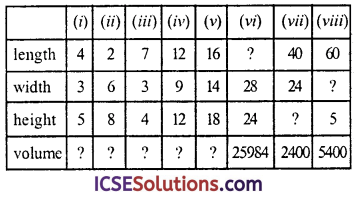Solution:
(i) Length = 4 cm, breadth = 3 cm and height = 5 cm
∴ Volume = lbh = 4 × 3 × 5 cm3 = 60 cm3

(ii) Length = 2 cm, width = 6 cm, height = 8 cm
∴ Volume = lbh = 2 × 6 × 8 cm3 = 96 cm3

(iii) Length = 7 cm, width = 3 cm and height = 4 cm
∴ Volume = lbh = 7 × 3 × 4 cm3 = 84 cm3

(iv) Length = 12 cm, width = 9 cm and height = 12 cm
∴ Volume = lbh = 12 × 9 × 12 cm3 = 1296 cm3

(v) Length = 16 cm, wdith = 14 cm and height = 18 cm
∴ Volume = lbh = 16 × 14 × 18 cm3 = 4032 cm3

(vi) Length = 7, width = 28 cm, and height = 24 cm
Volume = 25984 cm3
∴ Length = $$\frac{\text { Volume }}{b \times h}$$ = $$\frac{25984}{28 \times 24}$$ = 38.66 cm

(vii) Length = 40 cm, width = 24, volume = 2400 cm3
∴ height = $$\frac{\text { Volume }}{l \times b}$$ = $$\frac{2400}{40 \times 24}$$ = $$\frac { 5 }{ 2 }$$ = 2.5 cm

(viii) Length = 60 cm, height = 5 cm and volume = 5400 cm3
∴ Width = $$\frac{\text { Volume }}{l \times b}$$ = $$\frac{5400}{60 \times 5}$$ cm = 18 cm
Now the complete table is given below :Question 4.
Find the length of the diagonal of the cuboid whose dimensions are :
(a) 2, 3 and 4 cm
(b) 3, 4 and 5 cm
Solution:
(a) Dimension of a cuboid are 2,3 and 4 cm
∴ Its diagonal = $$\sqrt{l^2+b^2+h^2}$$
= $$\sqrt{(2)^2+(3)^2+(4)^2}$$
= $$\sqrt{4+9+16}$$ = $$\sqrt{29}$$ cm = 5.38 cm

(b) Dimensions of a cuboid are 3, 4, 5 cm
∴ Its diagonal = $$\sqrt{l^2+b^2+h^2}$$ = $$\sqrt{(3)^2+(4)^2+(5)^2}$$ = $$\sqrt{9+16+25}$$ = $$\sqrt{50}$$ = 7.07 cm

Question 5.
(i) Find the length of the diagonal and the volume of the cubes whose each edge is :
(a) 2 m,
(b) 5 m,
(c) 8 cm.
(ii) Find the volume and the total surface area of a cube if its edge is 12 cm.
Solution:
(i) (a) Edge of the cube (a) = 2 m
∴ Length of diagonal = $$\sqrt{3}$$ a
= $$\sqrt{3}$$ × 2 m = 2√3 m
= 2(1.732) = 3.464 m
and volume = a3 = (2)2 m3
= 2 × 2 × 2 = 8 m3

(b) Edge of the cube (a) = 5 m
∴ Length of diagonal = √3 a = √3 × 8 cm
= 5 (1.732) = 8.660 = 8.66 m
and volume = a3 = (5)3 = 5 × 5 × 5 m3
= 125 m3

(c) Edge of the cube (a) = 8 cm
∴ Length of diagonal = √3 a = √3 a × 8 cm
= 8 (1.732)
= 13.858 = 13.86 cm
and volume = a3 = (8)3 = 512 cm3

(ii) Edge of the cube (a) = 12 cm
∴ Volume = a3 = (12)3 cm3
= 1728 cm3
and total surface area = 6a2
= 6 × (12)2 cm2 = 6 × 144 cm2 = 864 cm2Question 6.
(i) Find each edge of the cubes whose volumes are :
(a) 216 m
(b) 2197 m3
The volume of a rectangular solid measuring 1 m by 50 cm by 0.5 m is 250000 cm3.
(iii) The diagonal of a rectangular solid is 5√2 dm. If its length and breadth are 5 dm and 4 dm, then find the height of the solid.
Solution:
(i) (a) Volume of a cube = 216 m3
∴ Ege = $$\sqrt{\text { Volume }}$$
= $$\sqrt{216}$$ = $$(6 \times 6 \times 6)^{\frac{1}{3}}$$
= $$\left(6^3\right)^{\frac{1}{3}}$$ = 6 m

(b) Volume of a cube = 2197 m3
∴ Ege = $$\sqrt{\text { Volume }}$$
$$\sqrt{2197}$$
= $$\left(13^3\right)^{\frac{1}{3}}$$ = 13 m

(ii) Length of cuboid (l) = 1 m = 100 cm
and height (h) = 0.5 m = $$\frac { 5 }{ 10 }$$ × 100 = 50 cm
Volume = lbh = 100 × 50 × 50 = 250000 cm3
Which is given
∴ It is true

(iii) Diagonal of rectangular solid = 5√2 dm
Let height be = h
Length (l) = 5 dm
and breadth (b) = 4 dm
∴ Diagonal = $$\sqrt{l^2+b^2+h^2}$$
⇒ 5√2 = $$\sqrt{(5)^2+(4)^2+h^2}$$
Squaring both sides,
50 = 25 + 16 + h2 ⇒ 50 = 41 + h2
h2 = 50 – 41 = 9 = (3)2
∴ h = 3
∴ Height = 3 dm

Question 7.
The volume of a rectangular solid is 3600 cm3. If it is 20 cm long and 9 cm high, find its width.
Solution:
Volume of the rectangular solid = 3600 cm3
Length (l) = 20 cm
Height (h) = 9 cm
∴Width = $$\frac{\text { Volume }}{l \times h}$$ = $$\frac{3600}{20 \times 9}$$ = 20 cm

Question 8.
The length and breadth of a rectangular solid are respectively 25 cm and 20 cm. If the volume is 7000 cm3, find its height.
Solution:
Length of rectangular solid (l) = 25 cm and breadth (b) = 20 cm
Volume = 7000 cm3
∴ Height $$\frac{\text { Volume }}{l \times b}$$ = $$\frac{7000}{25 \times 20}$$ cm

Question 9.
The perimeter of one face of a cube is 20 cm. Find (i) the total area of the 6 faces, (ii) the volume of the cube.
Solution:
Perimeter of one face of a cube = 20 cm
∴ Edge (side) (a) = $$\frac { 20 }{ 4 }$$ = 5 cm

(i) Total surface area = 6a2 = 6 × (5)3 cm2 = 6 × 25 = 150 cm2
(ii) Volume of cube = a3 = (5)3 = 5 × 5 × 5 cm3 = 125 cm3

Question 10.
The area of a playground is 4800 m2. Find the cost of covering it with gravel 1 cm deep, if the gravel costs Rs. 4.80 per cubic metre.
Solution:
Area of a playground = 4800 m2
Depth of gravel covered (h) = 1 cm = $$\frac { 1 }{ 100 }$$ m
∴ Volume = Area of the base × height = 4800 × $$\frac { 1 }{ 100 }$$ = 48 m3
Rate of graving = Rs. 4.80 pet cubic metre
∴ Total cost = Rs. 48 × 4.80 = Rs. 230.40

Question 11.
A rectangular water tank of base 7 m × 6 m contains water up to a depth of 5 m. How many cubic metres of water are there in the tank?
Solution:
Base of the tank = 7 m × 6 m
∴ Area of the base = 7 × 6 = 42 m2 Depth = 5 m
∴ Volume of the water in the tank
= Area × depth
=42 × 5 = 210 m3Question 12.
The internal measurement of a box are 20 cm long, 16 cm wide, and 24 cm high. How many $4 \mathrm{~cm}$ cubes could be put into the box?
Solution:
Internal length of box (l) = 20 cm
Width (b) = 16 cm
and height (h) = 24 cm
∴ Volume = l × b × h
= 20 × 16 × 24 = 7680 cm3
Side (Edge) of cube = 4 cm
Volume of one cube = (a)3 = (4)3 cm3 = 4 × 4 × 4 = 64 cm3
∴ No. of cubes kept in the box
= $$\frac{\text { Volume of box }}{\text { Volume of one cube }}$$
$$\frac { 7680 }{ 64 }$$ = 120

Question 13.
The length, breadth and height of a rectangular solid are in the ratio of 5 : 4 : 2. If the total surface area is 1216 cm2, find the length, breadth and height of the solid.
Solution:
Ratio in dimensions of the solid = 5 : 4 : 2
and surface area = 1216 cm3
Let length of rectangular solid (l) = 5x
and height (h) = 2x
Surface area = 2(lb + bh + hl)
∴ 2[5x × 4x + 4x × 2x + 2x × 5x] = 1216
⇒ 2 [20 x2 + 8x2 + 10x2] = 1216
⇒ 2 × 38x2 = 1216 ⇒ x2 = $$\frac{1216}{2 \times 38}$$
⇒ x2 = 16 = (4)2
∴ x = 4
∴ Length = 5x = 5 × 4 = 20 cm
Breadth = 4x = 4 × 4 = 16 cm
and height = 2x = 2 × 4 = 8 cm

Question 14.
A lock in a canal is 40 m long, 7 m wide. When the sluices are opened, the depth of water in the lock decreases from 5 m to 3 m 80 cm. How many cubic metre of water runs out?
Solution:
Length of lock of canal (l) = 40 m
Width (d) = 7 m
and level of water decreased (h) = 5 – 3.80 = 1.20 m
∴ Volume of water runs out = lbh
= 40 × 7 × 1.20 m3 = 336 m3

Question 15.
How many rectangular blocks, each 2$$\frac { 1 }{ 2 }$$cm by 1$$\frac { 1 }{ 2 }$$ cm can be packed in a box 90 cm by 78 cm by 42 cm, internal measurements? How many 4 cm cubes can be packed in this box, with faces parallel to the sides?
Solution:
Length of box (h) = 90 cm
Width (b) = 78 cm
Height (h) = 42 cm
∴ Volume = l × b × h = 90 × 78 × 42 cm3

(i) Length of rectangular block (l) = 2$$\frac { 1 }{ 2 }$$cm
Width (b) = 2 cm
and height (h) = 1$$\frac { 1 }{ 2 }$$ cm
∴ Volume of one block = lbh
= 2$$\frac { 1 }{ 2 }$$ × 2 × 1$$\frac { 1 }{ 2 }$$ cm3
= $$\frac { 5 }{ 2 }$$ × 2 × $$\frac { 3 }{ 2 }$$ cm3
= $$\frac { 15 }{ 2 }$$ cm3
∴ No. of block packed in the box
= $$\frac{\text { Volume of box }}{\text { Volume of one block }}$$
= $$\frac{98 \times 78 \times 42 \times 2}{15}$$ = 39312

(ii) Edge of one cube = 4 cm
∴ Volume = (4)3 = 4 × 4 × 4 = 64 cm2
∴ No. of cubes to be packed = $$\frac{90 \times 78 \times 42}{4}$$
Along length wise, no. of complete cubes
= $$\frac { 90 }{ 4 }$$ = 22
and along breadth wise, no. complete 4 cubes
= $$\frac { 78 }{ 4 }$$ = 19
and along height wise = no. of complete cubes
= $$\frac { 42 }{ 4 }$$ = 10
Total number of cubes = 22 × 19 × 10 = 4180

Question 16.
A tank 72 cm long, 60 cm wide, 36 cm high, contains water to a depth of 18 cm. A metal block 48 cm by 36 cm by 15 cm is put into the tank and totally submerged. Find in cm the amount the water level rises.
Solution:
Length of tank (l) = 72 cm
Width (b) = 60 cmand height (h) = 36 cm
Depth of water = 18 cm
Volume of water in the tank
=72 × 60 × 18 × cm3 = 77760 cm3
Length of block = 48 cm
and height = 15 cm
∴ Volume of block = 48 × 36 × 15 cm2 = 25920 cm3
∴ Height of water level rose up in the tank = $$\frac{25920}{72 \times 60}$$ = 6 cmQuestion 17.
Find the volume of wood required for making the closed box with internal dimensions : 20 cm by 12.5 cm by 9.5 cm; wood 1.25 cm thick.
Solution:
Internal length of the closed box (l) = 20 cm
and height (h) = 9.5 cm∴Inner volume = lbh
= 20 × 12.5 × 9.5 cm3 = 2375 cm3
Thickness of wood = 1.25 cm
∴ Outer length (L) = 20 + 2 × 1.25 cm
= 20 + 2.5 = 22.5 cm
Outer breadth (B) = 12.5 + 2 × 1.25 cm
= 12.5 + 2.5 = 15
and outer height (H) = 9.5 + 2 × 1.25 cm
= 9.5 + 2.5 = 12 cm
Outer volume = 22.5 × 15 × 12 cm2
= 4050 cm3
∴ Volume of wood used
= Outer volume – Inner volume
= 4050 – 2375 = 1675 cm3

Question 18.
Find the volume of wood required for making the open box with external dimensions 17.5 cm long. 14 cm wide, 10 cm high, wood 7.5 mm thick.
Solution:
In an open box
External length (L) = 17.5 cm
Height (H) = 10 cm
∴ Volume = LBH = 17.5 × 14 × 10 cm3
= 2450 cm3
Thickness of wood = 7.5 mm = 0.75 cm
∴ Inner length (l) = 17.5 – 2 × 7.5
=17.5 – 1.5 = 16 cm
Breadth (b) = 14 – 2 × .75
=14 – 1.5 = 12.5 cm
and height = 10 – 0.75 = 9.25 m ( ∵ box is open)
∴ Inner volume = 16 × 12.5 × 9.25 cm3 = 1850 cm3
∴ Volume of wood used = Outer volume – inner volume
=2450 – 1850 = 600 cm3

Question 19.
A field is 30 m long and 18 m broad. A pit 6 m long, 4 m wide and 3 m deep, is dug out from the middle of the field and the earth removed is evenly spread over the remaining area to the field, Find the rise in the level of the remaining part of the field in centimetres correct to one decimal place.
Solution:
Length of field (L) = 30 m
and breadth (B) = 18 m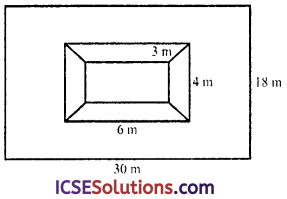Area = L × B
= 30 × 18 = 540 m2
Length of the pit (l) = 6 m
and depth (h) = 3 m
∴ Area of the face of the pit = l × b
=6 × 4 = 24 m2
and volume of the earth dugout
= lbh = 6 × 4 × 3 = 72 m3
Now area of the remaining field leaving the pit = 540 – 24 = 516 m2
∴ Height of the earth level
= $$\frac{\text { Volume of earth }}{\text { Area of the remaining field }}$$
= $$\frac { 72 }{ 516 }$$m
= $$\frac { 72 }{ 516 }$$ × 100cm = 13.9 cm (approx)

Question 20.
An agricultural field is in the form of a rectangle of length 20 m and width 14 m. A pit 6 m long, 3 m wide and 2.5 m deep is dug in the corner of the field and the earth taken out from the pit is spread uniformaly over the remaining area of the field. Find the extent to which the level of the field has been raised.
Solution:
Length of field (L) = 20 m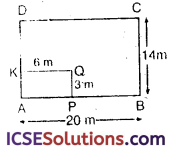∴ Area of the field = L × B
= 20 × 14 = 280 m2
Length of the pit (l) = 6 m
and depth (h) = 2.5 m
∴ Area of the face of the pit = l × b

= 6 × 3 = 18 m2
and volume of earth dugout = l.b.h
= 6 × 3 × 2.5 = 45 m3
∴ Area of the remanning field excluding the pit
=280 – 18 = 262 m2
∴ Height of the earth level
= $$\frac{\text { Volume of the earth }}{\text { Area of the remaining field }}$$
= $$\frac { 45 }{ 262 }$$ m = $$\frac{45 \times 100}{262}$$ cm
= 17.175 = 17.18 cm

Question 21.
A certain quality of wood costs Rs. 250 per m3. A solid cubical block of such wood is bought for Rs. 182.25. Calculate the volume of the block and use the method of factors to find the length of one edge of the block.
Solution:
Total cost of wood = Rs. 182.25
Rate of wood = Rs. 250 per m3
∴ Volume of the cubical block = $$\frac{182.25}{250}$$
= $$\frac{18225}{100 \times 250}$$ = 0.729 m3
= 729 × 100 × 100 × 100 = 729000 cm3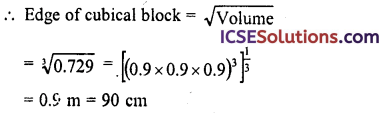Question 22.
A rectangular container, whose base is a square of side 6 cm, stands on a horizontal table and holds water upto 1 cm from the top. When a cube is placed in the water and is completely submerged, the water rises to the top and 2 cm3 of water overflows. Calculate the volume of the cube.
Solution:
In a rectangular container,
Side of the square base of the container = 6 cm
Water level = 1 cm from the top
On placing a cube in it, volume of water
= 6 × 6 × 1 cm3 + 2 cm3
= 36 + 2 = 38 cm3
∴ Volume of cube = 38 cm3

Question 23.
Two cubes, each with 8 cm edge are joined end to end. Find the surface area of the resulting cuboid.
Solution:
By joining two cubes of 8 cm edge the resulting cuboid will have
Length (l) = 8 + 8 = 16 cm
and height (h) = 8 cm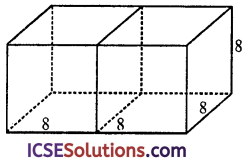∴ Surface area = 2(lb + bh + hl)
= 2 (16 × 8 + 8 × 8 + 8 × 16) cm2
= 2 (128 + 64 + 128) = 2 × 320 cm2
= 640 cm2Question 24.
The areas of three adjacent faces of a cuboid are x, y and z. If the volume is V, prove that V2 = xyz.
Solution:
Let length of the cuboid = l
and height = h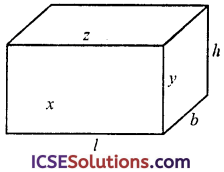∴ x = lh …(i)
y = bh …(ii)
z = lb …(iii)
and V = lbh …(iv)
Now xyz = lb × bh × lb [From (i, ii, iii)]
=l2 b2 h2 = (lbh)2
= V2 [From (iv)]
Hence proved.

Question 25.
A metal cube of edge 12 cm is melted and formed into three smaller cubes. If the edges of two smaller cubes are 6 cm and 8 cm, find an edge of the third smaller cube.
Solution:
Edge of metal cube (a) = 12 cm
∴ Volume = a3 = (12)3 cm3
=12 × 12 × 12 = 1728 cm3

On melting it, three smaller cubes are formed Edge of first smaller cube (a1) = 6 cm
∴ Volume = (a1)3 = (6)3 cm3
= 216 cm3

Edge of second smaller cube (a2)=8 cm
∴ Volume = (a2)3 = (8)3 cm3
= 512 cm3
∴ Volume of third smaller cube
= 1728 – (216 + 512)
= 1728 – 728 = 1000 cm3
∴ Edge of the third smaller cube
$$\sqrt{\text { Volume }}$$ = $$\sqrt{1000}$$ = $$\left(10^3\right)^{\frac{1}{3}}$$ = 10 cm

Question 26.
50 students sit in a class room. Each student requires 9 m2 on floor and 108 m3 in space. If the length of the room is 25 m, find its breadth and height.
Solution:
No. of students = 50
Area required for each student = 9 m2
∴ Total area of the floor = 50 × 9 m2 = 450 m2
Volume required for each student = 108 m3
∴ Total volume of air of the room
= 108 × 50 m3 = 5400 m3
Length of room = 25 m
∴ Breadth (b) = $$\frac{\text { Area of floor }}{\text { Length }}$$ = $$\frac { 450 }{ 25 }$$ = 18 m
and height = $$\frac{\text { Volume }}{l \times b}$$ = $$\frac{5400}{25 \times 18}$$ = 12 m
Hence breadth = 18 m and height = 12 m

Question 27.
A carboard sheet is of rectangular shape with dimensions 42 cm × 36 cm. From each one of its corner, a square of 6 cm is cut off. An open box is made of the remaining sheet. Find the volume of the box.
Solution:
Length of rectangular cardboard sheet (l) = 42 cmBy cutting squares from each corner, a box is formed whole length (l) = 42 – (2 × 6) = 42 – 12 = 30 cm
Breadth = 36 – 2 × 6 = 36 – 12 = 24 cm
and height (h) = 6 cm
∴ Volume = lbh = 30 × 24 × 6 cm3
= 4320 cm3

Question 28.
Two cubes, each of volume 343 cm3 are joined end to end. Find the surface area of the resulting cuboid.
Solution:
Volume of each of two cubes = 343 cm3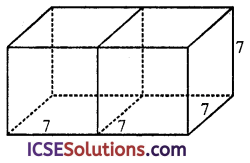∴ Edge of each cube = $$\sqrt{343}$$
= $$\left(7^3\right)^{\frac{1}{3}}$$ = 7 cm
Now by joining two cubes, a cuboid is formed, then
Length of cuboid (l) = 7 + 7 = 14 cm